top of page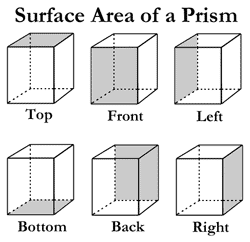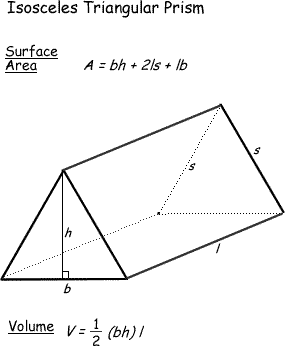## Volume & Surface Area

##### Surface Area  (Resources)
1. Find the Surface Area - Interactive

Explore solids to identify their suface area.

2. Rectangular Prisms - Surface Area

Notes and interactive models

3. Surface Area  Teacher Favorite

This site has it all, a lesson, interactive learning, questions and applications!

Surface Area of

##### Rectangular Prism (Games)
1. Surface Area Quiz

Scroll down to take the surface area quiz.

2. Another Surface Area Quiz

See how well you can do!

##### Volume (Resources)
1. BBC - Volume

Helps you to understand and see how the formula works!

2. Math is Fun

See examples of volume with rectangular prisms.

##### Volume (Games)
1. Calculate the Volume Quiz

Scroll down to take the quiz on volume.

Take this  5 question quiz to test your knowledge of volume.

3. Volume of Triangular Prisms

AAA Math finding the volume of a triangular prism.

4. Quia Volume of Triangular Prisms & Cylinders
Just skip the problems that involve cylinders.

5. Volume of Cubes & Rectangular Prisms IXL
Find the voume and test your knowledge.

6. Volume of a Rectangular Prism AAA Math
See how well you can do!

##### Volume and Surface Area (Resources)
1. Formulas for Area and Volume

Scroll down to identify the formulas.

2. Rectangular Prisms - Volume

Notes and interactive models to assess your understanding.

##### Volume and Surface Area (Games)
1. Calculate Volume and Surface Area

You can even choose the colors of your solids.

2. Find the Volume and Surface Area

Correctly identify the volume and surface area - test your knowledge.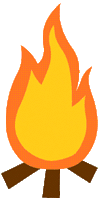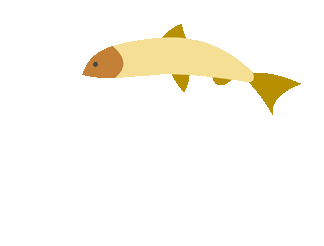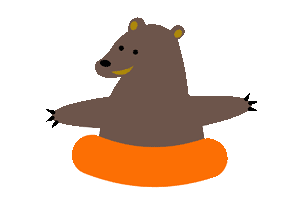bottom of page